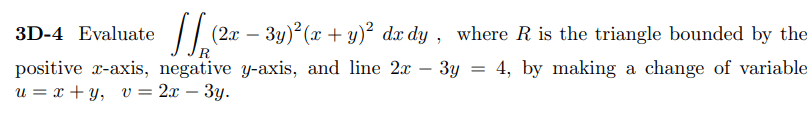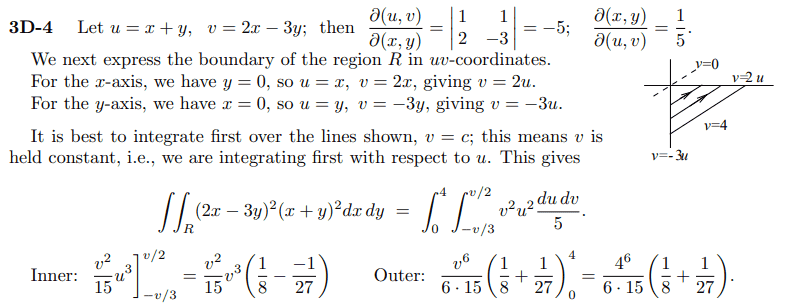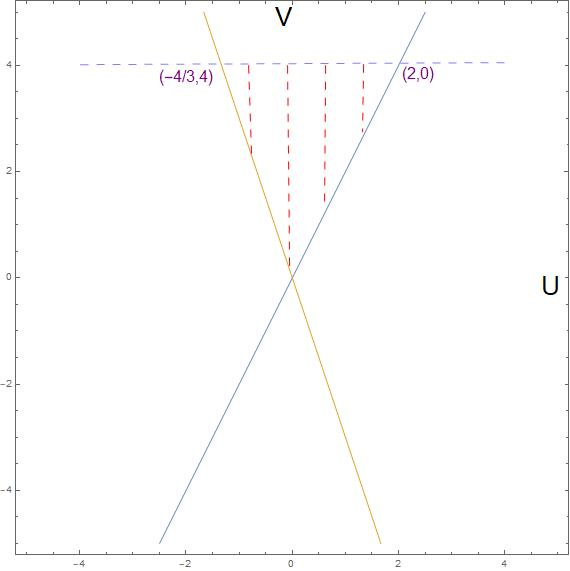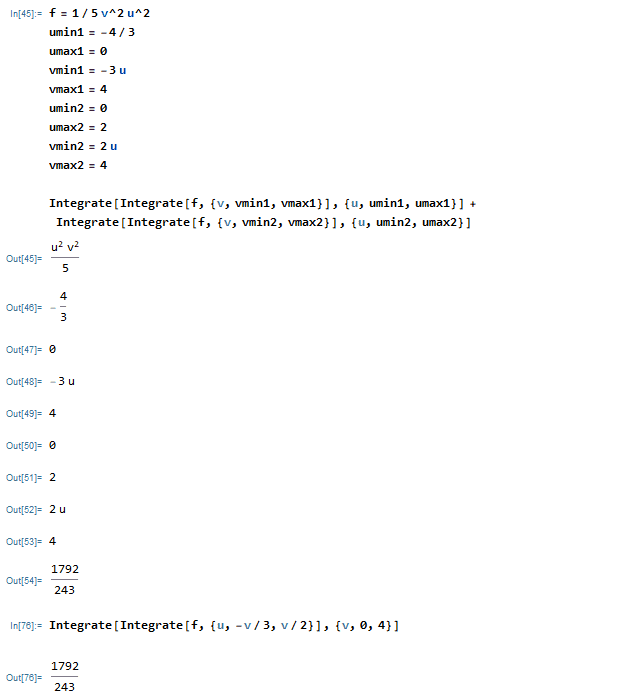# Find the bounds after changing the variables in a double integral

Leo Liu
Homework Statement:
.
Relevant Equations:
.The answer calculates the integral with ##du## before ##dv## as shown below.However I decided to compute it in the opposite order with different bounds. Here is my work:
According to the definitions, $$\begin{cases} u=x+y\\ v=2x-3y \end{cases}$$
First we need to convert the boundaries in xy into uv. The equation of y-axis is ##v=-3u## and the equation of x-axis is ##v=2u##. This two lines give the lower and upper limits of the inner integral dv. The bounds for u can be found by substituting ##x=0## and ##y=0## into ##2x-3y=4##. The lower bounds appears at the lower vertex of the triangle with the value of ##u=-4/3##. Similarly, the upper bounds is the right vertex at ##u=2##. After putting things together we get
$$\frac 1 5 \int _{-4/3}^{2}\int _{-3u}^{2u}v^2u^2\,dvdu$$
I'd like someone to check if the bounds in this integral are correct. Thanks in advance.

Homework Helper
Hi,

If you did everything correctly, your integral would yield the same as what the alternative yields. I get a different result, though.

Draw some lines where ##u## is constant and check the bounds of ##v##

##\ ##

•Leo Liu
Leo Liu
Hi,

If you did everything correctly, your integral would yield the same as what the alternative yields. I get a different result, though.

Draw some lines where ##u## is constant and check the bounds of ##v##
Thank you! I think I messed up the bounds for v.The graph above shows that heights of the endpoints of the line segment spanning the height of the triangle at a fixed x value are ##v=4## and ##v=-3u## or ##v=2u##; the choice of the lower bound depends on x. Hence the double integral can be written as

$$\frac 1 5 \int _{-4/3}^{0}\int _{-3u}^{4}v^2u^2\,dvdu+\frac 1 5 \int _{0}^{2}\int _{2u}^{4}v^2u^2\,dvdu$$
Is this correct?

Homework Helper
You can evaluate and check for yourself ...

##\ ##

•Leo Liu
Leo Liu
You can evaluate and check for yourself ...

##\ ##
I think I got it right. I appreciate your help.•BvU